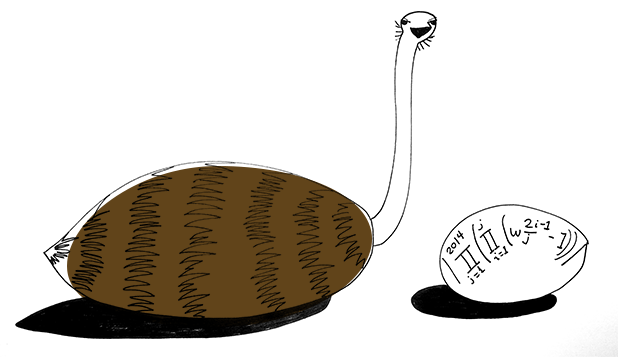# Expanding Seems Implausible

Algebra Level 5Let $\omega_n=e^{i\pi/n}$. The value of $\left|\prod_{j=1}^{2014}\left(\prod_{i=1}^j\left(\omega_j^{2i-1}-1\right)\right)\right|$ can be expressed as $a^b$ where $a,b$ are positive integers and $a$ is as small as possible. What is $a+b$?

×